Momentum and Its Conservation - Lesson 2 - The Law of Momentum Conservation

# The Law of Action-Reaction (Revisited)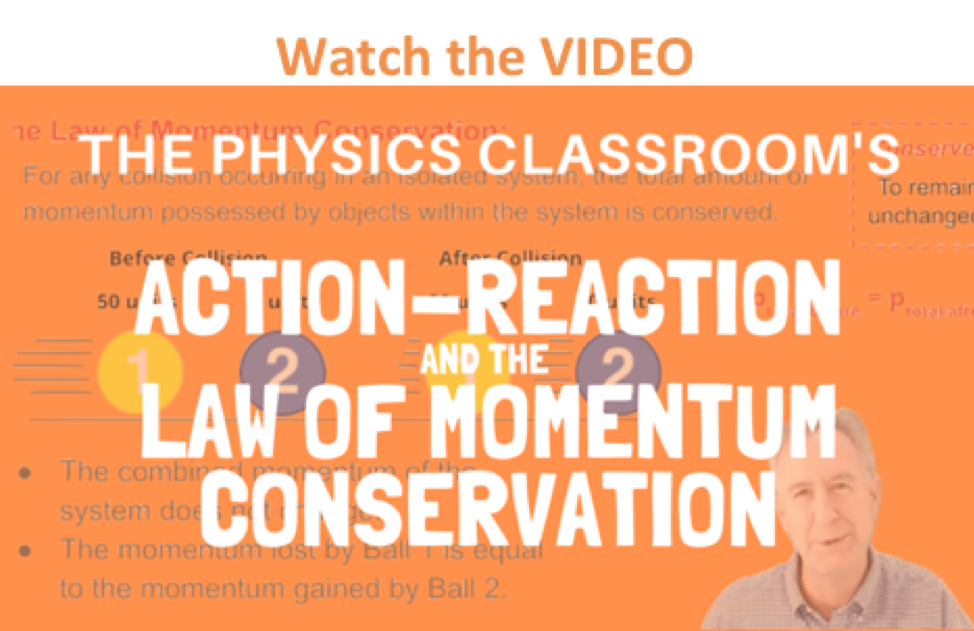A collision is an interaction between two objects that have made contact (usually) with each other. As in any interaction, a collision results in a force being applied to the two colliding objects. Newton's laws of motion govern such collisions. In the second unit of The Physics Classroom, Newton's third law of motion was introduced and discussed. It was said that...

... in every interaction, there is a pair of forces acting on the two interacting objects. The size of the force on the first object equals the size of the force on the second object. The direction of the force on the first object is opposite to the direction of the force on the second object. Forces always come in pairs - equal and opposite action-reaction force pairs.

###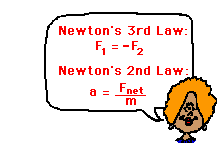Newton's Laws Applied to Collisions

Newton's third law of motion is naturally applied to collisions between two objects. In a collision between two objects, both objects experience forces that are equal in magnitude and opposite in direction. Such forces often cause one object to speed up (gain momentum) and the other object to slow down (lose momentum). According to Newton's third law, the forces on the two objects are equal in magnitude. While the forces are equal in magnitude and opposite in direction, the accelerations of the objects are not necessarily equal in magnitude. In accord with Newton's second law of motion, the acceleration of an object is dependent upon both force and mass. Thus, if the colliding objects have unequal mass, they will have unequal accelerations as a result of the contact force that results during the collision.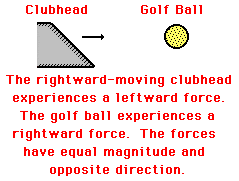Consider the collision between the club head and the golf ball in the sport of golf. When the club head of a moving golf club collides with a golf ball at rest upon a tee, the force experienced by the club head is equal to the force experienced by the golf ball. Most observers of this collision have difficulty with this concept because they perceive the high speed given to the ball as the result of the collision. They are not observing unequal forces upon the ball and club head, but rather unequal accelerations. Both club head and ball experience equal forces, yet the ball experiences a greater acceleration due to its smaller mass. In a collision, there is a force on both objects that causes an acceleration of both objects. The forces are equal in magnitude and opposite in direction, yet the least massive object receives the greatest acceleration.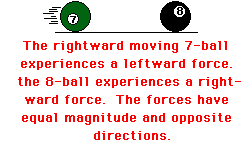Consider the collision between a moving seven ball and an eight ball that is at rest in the sport of table pool. When the seven ball collides with the eight ball, each ball experiences an equal force directed in opposite directions. The rightward moving seven ball experiences a leftward force that causes it to slow down; the eight ball experiences a rightward force that causes it to speed up. Since the two balls have equal masses, they will also experience equal accelerations. In a collision, there is a force on both objects that causes an acceleration of both objects; the forces are equal in magnitude and opposite in direction. For collisions between equal-mass objects, each object experiences the same acceleration.

Consider the interaction between a male and female figure skater in pair figure skating. A woman (m = 45 kg) is kneeling on the shoulders of a man (m = 70 kg); the pair is moving along the ice at 1.5 m/s. The man gracefully tosses the woman forward through the air and onto the ice. The woman receives the forward force and the man receives a backward force. The force on the man is equal in magnitude and opposite in direction to the force on the woman. Yet the acceleration of the woman is greater than the acceleration of the man due to the smaller mass of the woman.

Many observers of this interaction have difficulty believing that the man experienced a backward force. "After all," they might argue, "the man did not move backward." Such observers are presuming that forces cause motion. In their minds, a backward force on the male skater would cause a backward motion. This is a common misconception that has been addressed elsewhere in The Physics Classroom. Forces cause acceleration, not motion. The male figure skater experiences a backwards force that causes his backwards acceleration. The male skater slows down while the woman skater speeds up. In every interaction (with no exception), there are forces acting upon the two interacting objects that are equal in magnitude and opposite in direction.

Collisions are governed by Newton's laws. The law of action-reaction (Newton's third law) explains the nature of the forces between the two interacting objects. According to the law, the force exerted by object 1 upon object 2 is equal in magnitude and opposite in direction to the force exerted by object 2 upon object 1.

### Check Your Understanding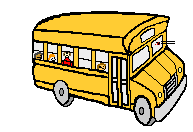Express your understanding of Newton's third law by answering the following questions. Click the button to check your answers.

1. While driving down the road, a firefly strikes the windshield of a bus and makes a quite obvious mess in front of the face of the driver. This is a clear case of Newton's third law of motion. The firefly hit the bus and the bus hits the firefly. Which of the two forces is greater: the force on the firefly or the force on the bus?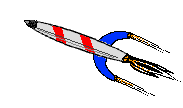2. For years, space travel was believed to be impossible because there was nothing that rockets could push off of in space in order to provide the propulsion necessary to accelerate. This inability of a rocket to provide propulsion in space is because ...

a. space is void of air so the rockets have nothing to push off of.

b. gravity is absent in space.

c. space is void of air and so there is no air resistance in space.

d. ... nonsense! Rockets do accelerate in space and have been able to do so for a long time.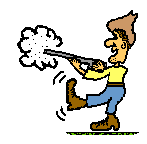3. Many people are familiar with the fact that a rifle recoils when fired. This recoil is the result of action-reaction force pairs. A gunpowder explosion creates hot gases that expand outward allowing the rifle to push forward on the bullet. Consistent with Newton's third law of motion, the bullet pushes backwards upon the rifle. The acceleration of the recoiling rifle is ...

a. greater than the acceleration of the bullet.

b. smaller than the acceleration of the bullet.

c. the same size as the acceleration of the bullet.

4. Kent Swimm, who is taking Physics for the third year in a row (and not because he likes it), has rowed his boat within three feet of the dock. Kent decides to jump onto the dock and turn around and dock his boat. Explain to Kent why this docking strategy is not a good strategy.

5. A clown is on the ice rink with a large medicine ball. If the clown throws the ball forward, then he is set into backwards motion with the same momentum as the ball's forward momentum. What would happen to the clown if he goes through the motion of throwing the ball without actually letting go of it? Explain.

6. Dale, Mel, and Shanelle are astronauts on a spaceship. They each have the same mass and the same strength. Dale and Mel decide to play catch with Shanelle, intending to throw her back and forth between them. Dale throws Shanelle to Mel and the game begins. Describe the motion of Dale, Mel and Shanelle as the game continues. If we assume that each throw involves the same amount of push, then how many throws will the game last?Next Section:

Follow Us